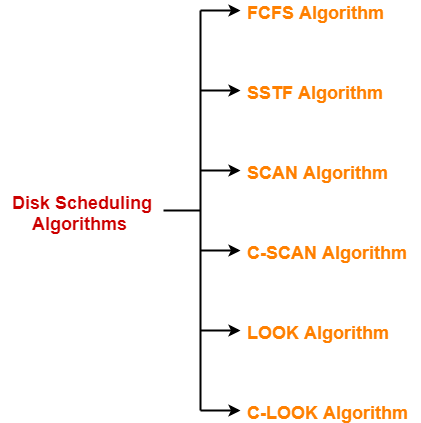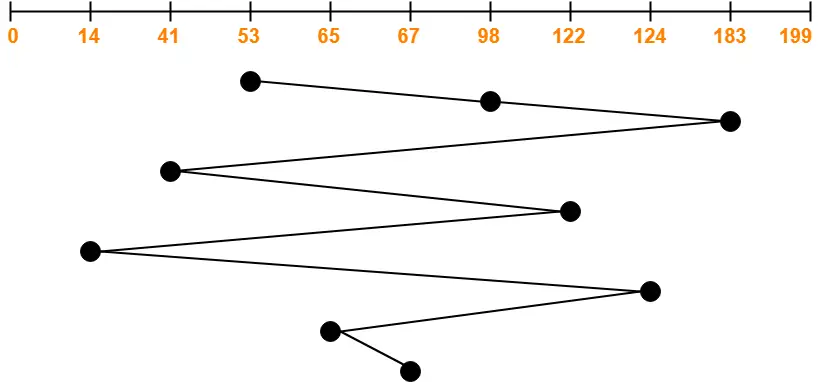# Disk Scheduling | Disk Scheduling Algorithms

## Disk Scheduling-

Before you go through this article, make sure that you have gone through the previous article on Magnetic Disk.

 Disk scheduling is a technique used by the operating system to schedule multiple requests for accessing the disk.

## Disk Scheduling Algorithms-

• The algorithms used for disk scheduling are called as disk scheduling algorithms.
• The purpose of disk scheduling algorithms is to reduce the total seek time.

Various disk scheduling algorithms are-1. FCFS Algorithm
2. SSTF Algorithm
3. SCAN Algorithm
4. C-SCAN Algorithm
5. LOOK Algorithm
6. C-LOOK Algorithm

## FCFS Disk Scheduling Algorithm-

• As the name suggests, this algorithm entertains requests in the order they arrive in the disk queue.
• It is the simplest disk scheduling algorithm.

• It is simple, easy to understand and implement.
• It does not cause starvation to any request.

• It results in increased total seek time.
• It is inefficient.

## Problem-

Consider a disk queue with requests for I/O to blocks on cylinders 98, 183, 41, 122, 14, 124, 65, 67. The FCFS scheduling algorithm is used. The head is initially at cylinder number 53. The cylinders are numbered from 0 to 199. The total head movement (in number of cylinders) incurred while servicing these requests is _______.

## Solution-Total head movements incurred while servicing these requests

= (98 – 53) + (183 – 98) + (183 – 41) + (122 – 41) + (122 – 14) + (124 – 14) + (124 – 65) + (67 – 65)

= 45 + 85 + 142 + 81 + 108 + 110 + 59 + 2

= 632

To gain better understanding about FCFS Disk Scheduling Algorithm,

Watch this Video Lecture

Next Article- SSTF Disk Scheduling Algorithm

Get more notes and other study material of Operating System.

Watch video lectures by visiting our YouTube channel LearnVidFun.

SummaryArticle Name
Disk Scheduling | Disk Scheduling Algorithms
Description
FCFS Disk Scheduling Algorithm is the simplest disk scheduling algorithm that services the requests on First Come First Serve basis. Example. The process which arrives first in the disk queue is entertained first.
Author
Publisher Name
Gate Vidyalay
Publisher Logo